• 《机器学习实战》5.2Logistic回归项目案例：预测病马死亡率 搜索微信公众号:‘AI-ming3526’或者’计算机视觉这件小事’ 获取更多人工智能、机器学习干货 csdn：https://blog.csdn.net/baidu_31657889/ github：...
《机器学习实战》5.2Logistic回归项目案例：预测病马死亡率

搜索微信公众号:‘AI-ming3526’或者’计算机视觉这件小事’ 获取更多人工智能、机器学习干货 csdn：https://blog.csdn.net/baidu_31657889/ github：https://github.com/aimi-cn/AILearners

本文出现的所有代码，均可在github上下载，不妨来个Star把谢谢~：Github代码地址
1、项目简介
本次实战内容，将使用Logistic回归来预测患疝气病的马的存活问题。原始数据集下载地址：数据集下载
这里的数据包含了368个样本和28个特征。这种病不一定源自马的肠胃问题，其他问题也可能引发马疝病。该数据集中包含了医院检测马疝病的一些指标，有的指标比较主观，有的指标难以测量，例如马的疼痛级别。另外需要说明的是，除了部分指标主观和难以测量外，该数据还存在一个问题，数据集中有30%的值是缺失的。下面将首先介绍如何处理数据集中的数据缺失问题，然后再利用Logistic回归和随机梯度上升算法来预测病马的生死。
2、开发流程
收集数据: 给定数据文件准备数据: 用 Python 解析文本文件并填充缺失值分析数据: 可视化并观察数据训练算法: 使用优化算法，找到最佳的系数测试算法: 为了量化回归的效果，需要观察错误率。根据错误率决定是否回退到训练阶段，通过改变迭代的次数和步长的参数来得到更好的回归系数使用算法: 实现一个简单的命令行程序来收集马的症状并输出预测结果并非难事，这可以作为留给大家的一道习题

收集数据: 给定数据文件

病马的训练数据已经给出来了，如下形式存储在文本文件中: 原始数据集地址
1.000000	1.000000	39.200000	88.000000	20.000000	0.000000	0.000000	4.000000	1.000000	3.000000	4.000000	2.000000	0.000000	0.000000	0.000000	4.000000	2.000000	50.000000	85.000000	2.000000	2.000000	0.000000
2.000000	1.000000	38.300000	40.000000	24.000000	1.000000	1.000000	3.000000	1.000000	3.000000	3.000000	1.000000	0.000000	0.000000	0.000000	1.000000	1.000000	33.000000	6.700000	0.000000	0.000000	1.000000


准备数据: 用 Python 解析文本文件并填充缺失值

数据中的缺失值是一个非常棘手的问题，很多文献都致力于解决这个问题。那么，数据缺失究竟带来了什么问题？假设有100个样本和20个特征，这些数据都是机器收集回来的。若机器上的某个传感器损坏导致一个特征无效时该怎么办？它们是否还可用？答案是肯定的。因为有时候数据相当昂贵，扔掉和重新获取都是不可取的，所以必须采用一些方法来解决这个问题。下面给出了一些可选的做法：
使用可用特征的均值来填补缺失值；使用特殊值来填补缺失值，如-1；忽略有缺失值的样本；使用相似样本的均值添补缺失值；使用另外的机器学习算法预测缺失值。
现在，我们对下一节要用的数据集进行预处理，使其可以顺利地使用分类算法。预处理数据做两件事：
如果测试集中一条数据的特征值已经确实，那么我们选择实数0来替换所有缺失值，因为本文使用Logistic回归。因此这样做不会影响回归系数的值。sigmoid(0)=0.5，即它对结果的预测不具有任何倾向性。如果测试集中一条数据的类别标签已经缺失，那么我们将该类别数据丢弃，因为类别标签与特征不同，很难确定采用某个合适的值来替换。
原始的数据集经过处理，保存为两个文件：horseColicTest.txt和horseColicTraining.txt。已经处理好的“干净”可用的数据集下载地址：
horseColicTraining.txt
horseColicTest.txt
有了这些数据集，我们只需要一个Logistic分类器，就可以利用该分类器来预测病马的生死问题了。

分析数据: 可视化并观察数据

将数据使用 MatPlotlib 打印出来，观察数据是否是我们想要的格式

训练算法: 使用优化算法，找到最佳的系数

我们会使用上一节用到的梯度上升和改进之后的随机梯度上升算法进行训练

测试算法: 为了量化回归的效果，需要观察错误率。根据错误率决定是否回退到训练阶段，通过改变迭代的次数和步长的参数来得到更好的回归系数

我们要做的就是使用Python构建Logistic回归分类器。使用Logistic回归方法进行分类并不需要做很多工作，所需做的只是把测试集上每个特征向量乘以最优化方法得来的回归系数，再将乘积结果求和，最后输入到Sigmoid函数中即可。如果对应的Sigmoid值大于0.5就预测类别标签为1，否则为0。
我们先使用梯度上升算法计算错误率大小 然后使用改进的随机梯度上升算法计算错误率大小
代码如下：
#!/usr/bin/env python
# -*- encoding: utf-8 -*-
'''
@File    :   Logistic_demo01.py
@Time    :   2019/05/27 16:31:12
@Author  :   xiao ming
@Version :   1.0
@Contact :   xiaoming3526@gmail.com
@Desc    :   Logistic回归案例之预测病马死亡率
@github  :   https://github.com/aimi-cn/AILearners
'''

# here put the import lib
import numpy as np
import random

'''
@description: sigmoid函数
@param  inX - 数据
@return: sigmoid函数
'''
def sigmoid(inX):
return 1.0 / (1 + np.exp(-inX))

'''
@description: 梯度上升法
@param {type} 两个参数：
第一个参数==> dataMatIn 是一个2维NumPy数组，每列分别代表每个不同的特征，每行则代表每个训练样本。
第二个参数==> classLabels 是类别标签，它是一个 1*100 的行向量。为了便于矩阵计算，需要将该行向量转换为列向量，做法是将原向量转置，再将它赋值给labelMat。
@return: 求得的权重数组(最优参数)
'''
#用dataMatIn创建特征矩阵
dataMatrix = np.mat(dataMatIn)
#调换矩阵的坐标顺序，对于二维矩阵来说，transpose()就是转置
labelMat = np.mat(classLabels).transpose()
#m是样本数，n是特征数
m, n = np.shape(dataMatrix)
#梯度上升步长
alpha = 0.001
#最大迭代次数
maxCycles = 500
#权重向量b，初始化为全1 这里面n为3
weights = np.ones((n,1))
for k in range(maxCycles):
#讲给定的值通过sigmoid函数输出为0-1之间的数值 #对w1*x1+w2*x2求对数几率回归
h = sigmoid(dataMatrix * weights)
#计算真实值和预测值之间的误差
error = labelMat - h
#根据误差进行梯度更新
weights = weights + alpha * dataMatrix.transpose() * error
#.getA()将自身矩阵变量转化为ndarray类型的变量
return weights

'''
@description: 改进的随机梯度上升算法
@param  dataMatrix - 数据数组
classLabels - 数据标签
numIter - 迭代次数
@return: weights - 求得的回归系数数组(最优参数)
'''
#返回dataMatrix的大小。m为行数,n为列数。
m,n = np.shape(dataMatrix)
#参数初始化
weights = np.ones(n)
# 随机梯度, 循环150,观察是否收敛
for j in range(numIter):
# [0, 1, 2 .. m-1]
dataIndex = list(range(m))
for i in range(m):
# i和j的不断增大，导致alpha的值不断减少，但是不为0
alpha = 4/(1.0+j+i)+0.0001
# 随机产生一个 0～len()之间的一个值
# random.uniform(x, y) 方法将随机生成下一个实数，它在[x,y]范围内,x是这个范围内的最小值，y是这个范围内的最大值。
randIndex = int(np.random.uniform(0,len(dataIndex)))
# sum(dataMatrix[i]*weights)为了求 f(x)的值， f(x)=a1*x1+b2*x2+..+nn*xn
h = sigmoid(sum(dataMatrix[randIndex]*weights))
error = classLabels[randIndex] - h
#更新回归系数
weights = weights + alpha * error * dataMatrix[randIndex]
#删除已经使用的样本
del(dataIndex[randIndex])
return weights

def colicTest():
trainingSet = []; trainingLabels = []
currLine = line.strip().split('\t')
lineArr = []
for i in range(len(currLine)-1):
lineArr.append(float(currLine[i]))
trainingSet.append(lineArr)
trainingLabels.append(float(currLine[-1]))
#使用梯度上升训练
errorCount = 0; numTestVec = 0.0
numTestVec += 1.0
currLine = line.strip().split('\t')
lineArr =[]
for i in range(len(currLine)-1):
lineArr.append(float(currLine[i]))
if int(classifyVector(np.array(lineArr), trainWeights[:,0]))!= int(currLine[-1]):
errorCount += 1
errorRate = (float(errorCount)/numTestVec) * 100                                 #错误率计算
print("梯度上升算法测试集错误率为: %.2f%%" % errorRate).decode('utf-8').encode('gb2312')

def colicTest1():
trainingSet = []; trainingLabels = []
currLine = line.strip().split('\t')
lineArr = []
for i in range(len(currLine)-1):
lineArr.append(float(currLine[i]))
trainingSet.append(lineArr)
trainingLabels.append(float(currLine[-1]))
#使用改进的随机上升梯度训练
errorCount = 0; numTestVec = 0.0
numTestVec += 1.0
currLine = line.strip().split('\t')
lineArr =[]
for i in range(len(currLine)-1):
lineArr.append(float(currLine[i]))
if int(classifyVector(np.array(lineArr), trainWeights))!= int(currLine[-1]):
errorCount += 1
errorRate = (float(errorCount)/numTestVec) * 100                                 #错误率计算
print("随机梯度上升测试集错误率为: %.2f%%" % errorRate).decode('utf-8').encode('gb2312')

def classifyVector(inX, weights):
prob = sigmoid(sum(inX*weights))
if prob > 0.5: return 1.0
else: return 0.0

if __name__ == '__main__':
#使用梯度上升训练
colicTest()
#使用随机梯度上升训练
colicTest1()

运行结果如下：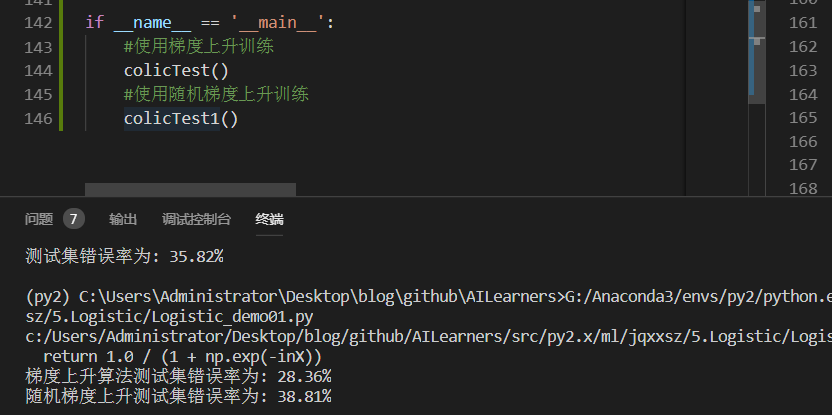随机梯度上升错误率还是蛮高的并且每次运行的错误率也是不同的，错误率高的时候可能达到40%多。为啥这样？首先，因为数据集本身有30%的数据缺失，这个是不能避免的。另一个主要原因是，我们使用的是改进的随机梯度上升算法，因为数据集本身就很小，就几百的数据量。用改进的随机梯度上升算法显然不合适。
我们看到梯度上升算法错误率稳定且较低。很显然，使用随机梯度上升算法，反而得不偿失了。
所以可以得到如下结论：
当数据集较小时，我们使用梯度上升算法当数据集较大时，我们使用改进的随机梯度上升算法

使用算法: 实现一个简单的命令行程序来收集马的症状并输出预测结果并非难事，这可以作为留给大家的一道习题

补充：对于这么复杂的分类算法 以及之后要学习的支持向量机SVM 都是非常复杂的 是不是很害怕自己写不出来代码 不用担心 这些东西其他开发者都为我们想好了 我们直接调用api就可以了 我们最好是了解一下他的原理 具体实现细节不会也无妨 我这里准备了使用sklearn实现Logistic回归的代码 核心代码只有两行就可以实现这样的算法 有兴趣的小伙伴去sklearn官网查看
sklearn.linear_model模块提供了很多模型供我们使用，比如Logistic回归、Lasso回归、贝叶斯脊回归等，可见需要学习的东西还有很多很多。
我使用了liblinear和sag方法进行实现
完整代码地址：https://github.com/aimi-cn/AILearners/tree/master/src/py2.x/ml/jqxxsz/5.Logistic/Logistic_demo02.py
liblinear(使用了开源的liblinear库实现，内部使用了坐标轴下降法来迭代优化损失函数)
sag方法(即随机平均梯度下降，是梯度下降法的变种，和普通梯度下降法的区别是每次迭代仅仅用一部分的样本来计算梯度，适合于样本数据多的时候。)
核心代码(两行)：
    # 使用solver优化算法选择参数，只有五个可选参数 我们使用liblinear和sag进行优化
# max_iter：算法收敛最大迭代次数，int类型，默认为10
classifier = LogisticRegression(solver='liblinear',max_iter=10).fit(trainingSet, trainingLabels)
# 此时max_iter=3000是因为10的话算法还没有收敛 所以就选择较大的数进行收敛
classifier2 = LogisticRegression(solver='sag',max_iter=3000).fit(trainingSet, trainingLabels)
# score方法返回测试之后的正确率
test_accurcy = classifier.score(testSet, testLabels) * 100
print("正确率为: %.2f%%" % test_accurcy).decode('utf-8').encode('gb2312')

运行结果如下：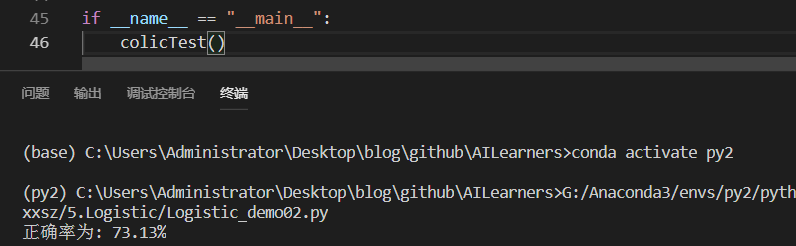优化的正确率达到7成以上 还是那句话，我们需要根据数据集情况，选择最优化算法。
对于每个函数的具体使用，可以看下官方文档：点我查看
AIMI-CN AI学习交流群【1015286623】 获取更多AI资料 扫码加群：分享技术，乐享生活：我们的公众号计算机视觉这件小事每周推送“AI”系列资讯类文章，欢迎您的关注！展开全文机器学习实战
• 爱彼迎 Airbnb价格预测-监督学习-回归项目
• 项目是数据的回归预测，针对混凝土抗压强度数据集Concrete_Data进行样本的混凝土抗压强度预测。先对特征之间，特征与label之间的一致性进行观察，然后选取一致性较高的特征进行分析，基于R^2指标，分别用线性回归/...
本项目是数据的回归预测，针对混凝土抗压强度数据集Concrete_Data进行样本的混凝土抗压强度预测。先对特征之间，特征与label之间的一致性进行观察，然后选取一致性较高的特征进行分析，基于R^2指标，分别用线性回归/岭回归/Lasso回归/ElasticNet/梯度boosting回归/支持向量机对数据集做单变量与多变量的回归预测分析。 src="https://nbviewer.jupyter.org/github/wzy6642/Machine-Learning-Case/blob/master/concrete/code/Concrete.ipynb" width="100%" height="1000">
github:https://github.com/wzy6642/Machine-Learning-Case
展开全文机器学习 Python 数据挖掘
• 机器学习回归预测Introduction: The applications of machine learning range from games to autonomous vehicles; one very interesting application is with education. With machine learning, regression ...

机器学习回归预测

Introduction: The applications of machine learning range from games to autonomous vehicles; one very interesting application is with education. With machine learning, regression algorithms, we can use a student dataset to predict the grades of students in their exams. This is an interesting application as it allows the teachers to be able to predict students grades early before the exams and find ways to assist the students who are not expected to perform so well. This article provides a detail explanation of how to use python to carry out this machine learning prediction task.
介绍： 机器学习的应用范围从游戏到自动驾驶汽车； 一个非常有趣的应用是教育。 通过机器学习，回归算法，我们可以使用学生数据集来预测学生在考试中的成绩。 这是一个有趣的应用程序，它使教师能够在考试前及早预测学生的成绩，并找到方法来帮助那些表现不佳的学生。 本文详细说明了如何使用python来执行此机器学习预测任务。
Dataset: This study considers data collected during the 2005–2006 school year from two public schools, from the Alentemol region of Portugal. The database was built from two sources: school reports, and questionnaires, related to several demographic (.e.g. Mother’s education, family income), social/emotional (e.g. alcohol consumption) and school related (e.g. number of past class failures) variables that are expected to affect student performance.
资料集： 本研究考虑了2005-2006学年期间从葡萄牙阿连特莫尔地区的两所公立学校收集的数据。 该数据库由两个来源建立：学校报告和调查表，与以下几个人口统计指标(例如母亲的学历，家庭收入)，社会/情感(例如饮酒)和学校相关(例如上课失败的次数)相关预期会影响学生的表现。
The datasets used for this project is publicly available on Kaggle and can be downloaded with these urls:
该项目使用的数据集可在Kaggle上公开获得，并可使用以下网址下载：
- https://www.kaggle.com/ozberkgunes/makineogrenmesiodev2-student-grande-prediction/data
-https://www.kaggle.com/ozberkgunes/makineogrenmesiodev2-student-grande-prediction/data
- https://www.kaggle.com/imkrkannan/student-performance-data-set-y-uci
-https://www.kaggle.com/imkrkannan/student-performance-data-set-y-uci
The dimensions in the dataset are all explained and summarized in the table below.
下表中解释并总结了数据集中的维度。
Data Pre-processing: Before we can apply our regression algorithms on our dataset, we first need to pre-process our dataset to make sure we have handled both empty and categorical values.
数据预处理： 在将回归算法应用于数据集之前，我们首先需要对数据集进行预处理，以确保我们处理了空值和分类值。
Firstly, we check for the empty values in our dataset by using the isnull and sum function as shown in the code snippet below.
首先，如下面的代码片段所示，我们使用notull和sum函数检查数据集中的空值。
Code snippet for reading dataset and checking for null values

We find out we only have 1 empty values for each column; we figure out we have an insignificant number of empty rows, hence we simply drop all empty columns by using the dropna function.
我们发现每一列只有1个空值； 我们发现空行的数量微不足道，因此我们只需使用dropna函数删除所有空列。
Code Snippet for dropping all null columns
用于删除所有空列的代码段

Selecting the Columns to Use for Regression Using Correlation:There are many ways to select the columns for regression; some of these ways include using p values, using their correlation, or using a feature selection method. In this case, our target column is G3 (the final exam result for the students), we decided to make use of a heatmap showing the correlation between all columns.
使用相关性选择要用于回归的列： 有很多方法可以选择要回归的列。 其中一些方法包括使用p值，使用它们的相关性或使用特征选择方法。 在这种情况下，我们的目标列是G3(学生的期末考试成绩)，我们决定使用显示所有列之间相关性的热图。
Code snippet to create correlation heat map

From the heat map we find that the columns with the most relevant correlation to G3 are G1,G2, Medu and failures and hence these are the columns we will use for our regression. G1 and G2 represent the student’s performance in previous assessments. This is not surprising as we would expect students who perform well to most likely perform well, again, in the final assessment. Medu represents the mothers level of education, while failures represents the number of assessments previously failed by the student. Both these two properties are not surprisingly as anyone would expect a student with very few previously failed courses to do well in an exam.
从热图中，我们发现与G3最相关的列是G1，G2，Medu和failures ，因此这些是我们将用于回归的列。 G1和G2代表学生在以前的评估中的表现。 这并不奇怪，因为我们期望表现良好的学生在最终评估中再次表现出色。 Medu代表母亲的教育程度，而失败则代表学生先前未通过的评估数量。 这两个属性都不令人惊讶，因为任何人都希望以前很少通过课程的学生在考试中表现出色。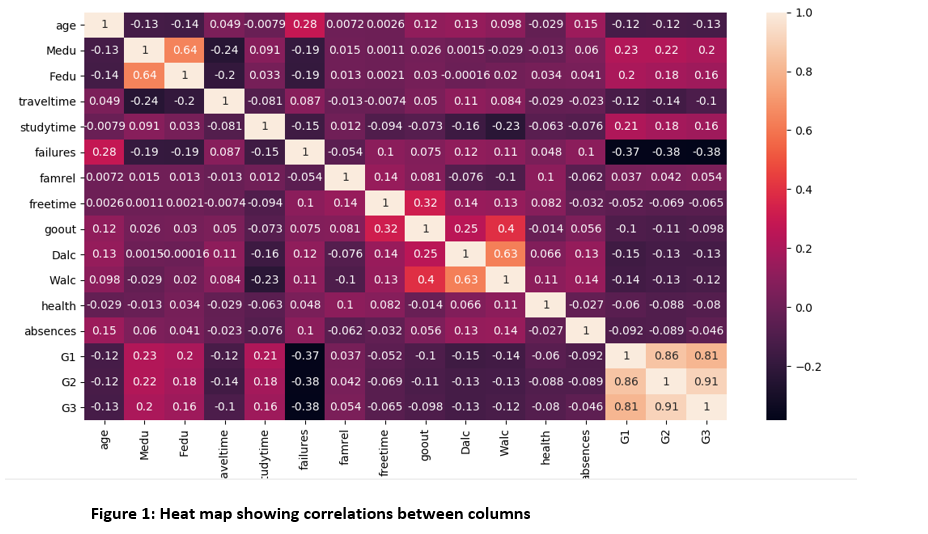Applying Regression Algorithm:The selected columns are all non-categorical values so no need to use any method i.e. one hot encoding to handle categorical data hence we can move straight to applying our regression algorithm on our dataset with our selected columns (G1,G2, Medu and failures).
应用回归算法： 选定的列都是非分类值，因此无需使用任何方法，即使用一种热编码来处理分类数据，因此我们可以直接将回归算法应用到具有选定列( G1，G2，Medu和failures )的数据集上。
I created a function to run the regression models, it accepts the algorithms names as parameter along with the regression objects and prints out the resulting model’s accuracy and RMSE.
我创建了一个运行回归模型的函数，它接受算法名称作为参数以及回归对象，并打印出结果模型的准确性和RMSE。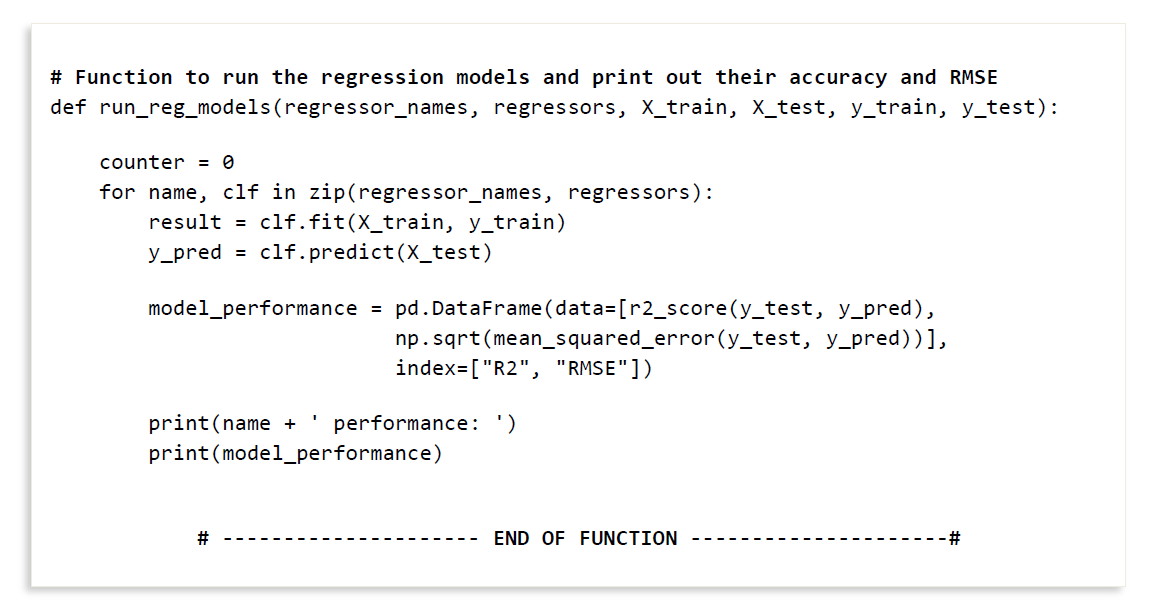For this project I selected 5 different regression algorithms to use on the dataset:
对于此项目，我选择了5种不同的回归算法以用于数据集：
- Linear regression
-线性回归
- Ridge regression
-岭回归
- Lasso regression
-套索回归
- Elastic Net regression
-弹性净回归
- Orthogonal Matching Pursuit CV regression
-正交匹配追踪CV回归
Before proceeding to training our regression models, we need to split our dataset into the training and testing data. This is very important as we don’t want to train and test our model with the same set of data hence the need for the split. We achieve this we the code snippet below:
在继续训练回归模型之前，我们需要将数据集分为训练和测试数据。 这一点非常重要，因为我们不想使用相同的数据集来训练和测试模型，因此不需要拆分。 我们通过以下代码片段实现了这一目标：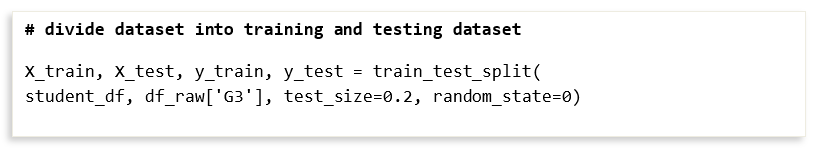We simply create an array of these regression models and pass it to the run _reg_models function as shown in the code snippet below:
我们只需创建一个这些回归模型的数组，然后将其传递给run _reg_models函数，如下面的代码片段所示：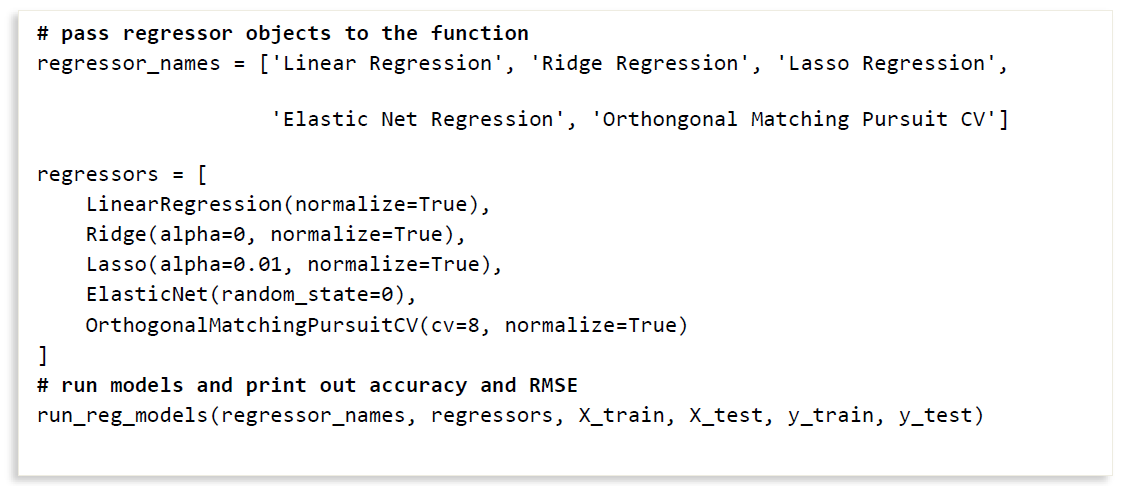Results and Future Works:
结果和未来工作：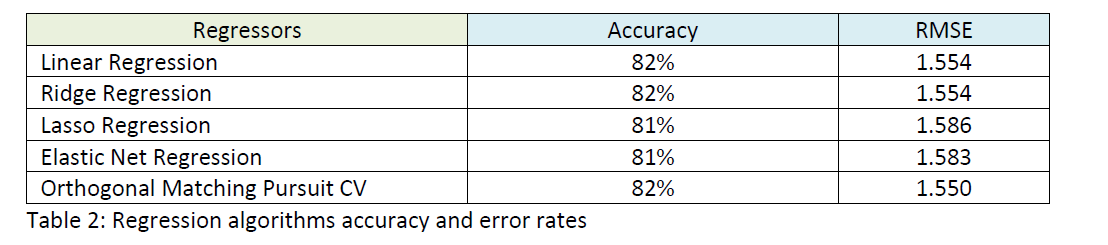The table above shows the resulting accuracies for the different regressor models with Linear, ridge and Orthogonal matching pursuit CV having the highest accuracy of 82% and the others at 81%. There is not a lot of difference between the different regression models both with their accuracy and RMSE.
上表显示了具有线性，脊形和正交匹配追踪CV的不同回归模型的最终精度，其最高精度为82％，其他精度为81％。 不同的回归模型在准确性和均方根误差方面没有太大差异。
An accuracy of 82% is okay but we would still need to fine tune the hyper-parameters i.e. test with different parameters for the different regression algorithms. If we do this, we may get higher accuracy values. It’s also important to note that this is not the final accuracy, we would still need to test our model(s) with external datasets to see how robust our model is.
82％的精度是可以的，但是我们仍然需要微调超参数，即针对不同的回归算法使用不同的参数进行测试。 如果这样做，我们可能会获得更高的精度值。 同样重要的是要注意，这不是最终的准确性，我们仍然需要使用外部数据集测试模型，以查看模型的健壮性。
There are many ways to select a model i.e. time to train model, time to predict and many other methods, but in this case we will use the model with the least RMSE as they all have similar accuracies and all take a similar time to run.
选择模型的方法有很多，例如训练模型的时间，预测的时间和许多其他方法，但是在这种情况下，我们将使用具有最小RMSE的模型，因为它们具有相似的精度并且都需要相似的时间来运行。
Our selected model is the Orthogonal pursuit as it has the lowest RMSE. In the future, we will play around with the hyperparameters in order to see how much of a difference it makes with both the accuracy and RMSE and if it makes a high difference, we will use redo our model selection.
我们选择的模型是正交追求，因为它具有最低的RMSE。 将来，我们将研究超参数，以了解它对精度和均方根误差的影响有多大，如果影响很大，我们将使用重做模型选择。
A future work will be to test our models with an external dataset (any dataset like this but one that our model has not been trained with before) and see how well our model performs. It is also important to note that the dataset used in this article was from a study carried out 15 years ago, another possible future works will be to find a newer dataset and to test our model on it and see the result.
未来的工作将是使用外部数据集(这样的任何数据集，但我们以前从未对其进行过训练的数据集)来测试我们的模型，并查看模型的性能如何。 还需要注意的是，本文中使用的数据集来自15年前进行的一项研究，未来的另一项可能的工作是找到一个更新的数据集并在其上测试我们的模型并查看结果。
Extra: Using A Model For Prediction
附加：使用模型进行预测
It’s interesting that most articles don’t include a section where they show case the prediction abilities of the model. Here, I have added this section so anyone who is a bit confused understands exactly how to use our models for predictions. Our selected model is the Orthogonal Matching Pursuit CV and hence it is what we use for the prediction as shown with the code snippet below:
有趣的是，大多数文章都没有包含展示模型预测能力的章节。 在这里，我添加了本节，以便任何有点困惑的人都能准确地了解如何使用我们的模型进行预测。 我们选择的模型是Orthogonal Matching Pursuit CV，因此它是我们用于预测的模型，如下面的代码片段所示：
Code snippet for using our selected model for making predictions

Our regressor isn’t a 100% accurate but it’s pretty close and hence could be really used to predict the grades of students quite well.
我们的回归指标并不是100％准确的，但它非常接近，因此可以真正用来很好地预测学生的成绩。
It’s possible to use the other models to also make predictions and it’s something you might be interested in trying out as an extra exercise.
可以使用其他模型进行预测，这可能是您有兴趣尝试做为额外练习的事情。
Thank you for reading my article, please reach out to me if you have any questions to ask.
感谢您阅读我的文章，如果您有任何疑问请与我联系。

机器学习回归预测

展开全文机器学习 人工智能 python 深度学习
• 使用sklearn的线性回归模型预测房价 我们已经留意到卧室数量属性信息有缺省，因此，我们先用该列的中位数填充这些缺省值。然后用DataFrame.isnull().sum()来统计缺省值的总数。我们用sklearn自带的评分函数lr.score...
1. 查看数据的基本信息
import numpy as np
import pandas as pd
import matplotlib.pyplot as plt
import seaborn as sns

path = r"D:\python\course\Aurelien Geron\housing.xlsx"
print(data.shape)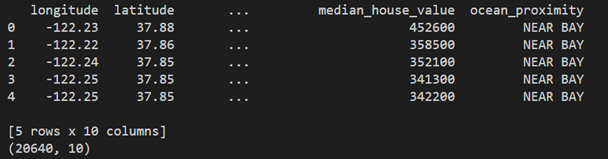print(data.info())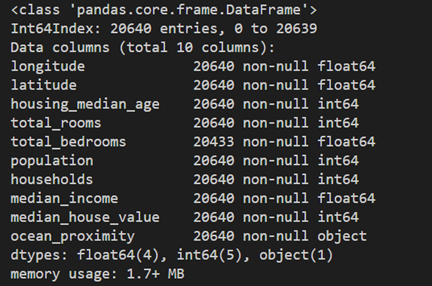从上图可以看到总登记房屋消息有20640条，而总卧室数量的条目数比其他项目少了7条，说明有7行空缺。
# 查看一下每列数据中有多少缺省值
print(data.isnull().sum(axis=0))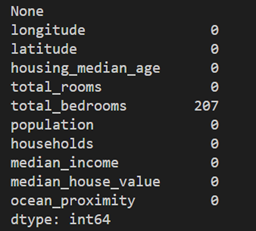2. 根据data.corr() 绘制heatmap
import numpy as np
import pandas as pd
import matplotlib.pyplot as plt
import seaborn as sns

path = r"D:\python\course\Aurelien Geron\housing.xlsx"

# 查找关联度, corr()函数会自动忽略掉字符串列“ocean_proximity”
corr_matrix = data.corr()
print(corr_matrix)

plt.figure(figsize=(15, 10))
ax = sns.heatmap(data.corr(), cmap=plt.cm.RdYlGn, annot=True, fmt=".2f", annot_kws={'size':18})

plt.legend(prop={"size":18})
# 设置刻度字体大小
plt.xticks(fontsize=18)
plt.yticks(fontsize=18)
# 可手动调整y轴的范围来进行修复。(老版本的Matplotlib不需要调整y轴范围。)
a, b = ax.get_ylim()
ax.set_ylim(a + 0.5, b - 0.5)
plt.show()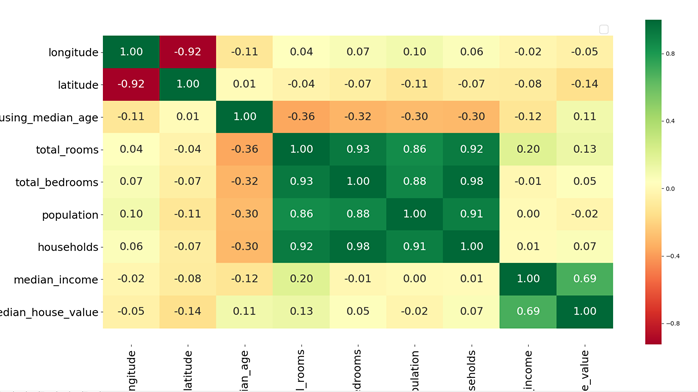从这个heatmap上可以看出来：
人口和家庭数的Pearson相关系数高度相关（达到0.98）；而总的卧室数目和家庭数目的Pearson相关系数也高度相关（高达0.88）；人口和总房间数的相关系数的正相关性也挺高（0.86）；而最关心的房价中位数和收入中位数的相关系数最高（0.69）。
因此我们想重点看一年房价中位数和收入中位数间更详细的关系。
先使用不设置alpha值的散点图查看房价中位数和收入中位数的关系（左图），可以看到散点太稠密，堆叠在一起，很看看清楚细节。而右边的图将alpha值设为0.1后，可以看到收入中位数和房价中位数间更加细致的对应关系。而pyplot 中的参数alpha 是负责颜色透明度的，值介于[0, 1]: 1表示不透明，0表示透明。
data.plot(kind='scatter', x='median_income', y='median_house_value')
plt.axis([0, 16, 0, 550000])
plt.show()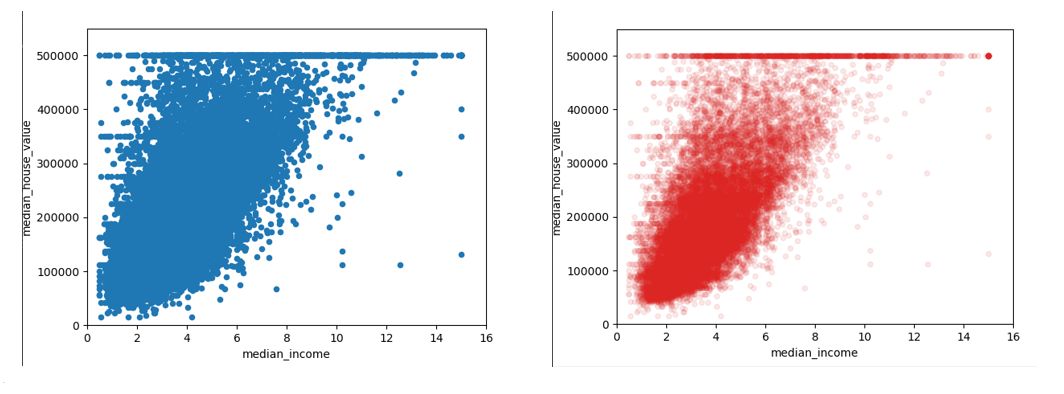data.plot(kind='scatter', x='median_income', y='median_house_value',
color="#dc2624", alpha=0.1)
plt.axis([0, 16, 0, 550000])
plt.show() 
4. 查看关键数据的分布形状
print(data.describe())
print(data["median_income"].skew())
print(data["median_house_value"].skew())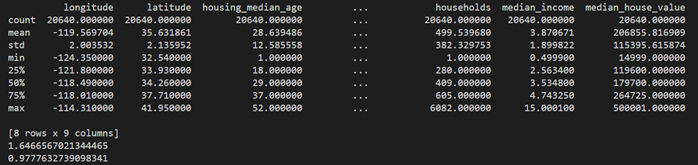从pd.Series.skew() 函数能计算得到收入中位数的偏度为1.646，而房产价格中位数的偏度为0.977。说明这两个数据组都有不同程度的右偏。而使用seaborn.displot()函数绘制的图像也能看到这一点。
plt.figure(figsize=(12, 5), dpi=80)
#第一行第一列图形
ax1 = plt.subplot(1,2,1)
#第一行第二列图形
ax2 = plt.subplot(1,2,2)

#选择ax1
plt.sca(ax1)
sns.distplot(data["median_income"].dropna())
#选择ax2
plt.sca(ax2)
sns.distplot(data["median_house_value"].dropna())
plt.show()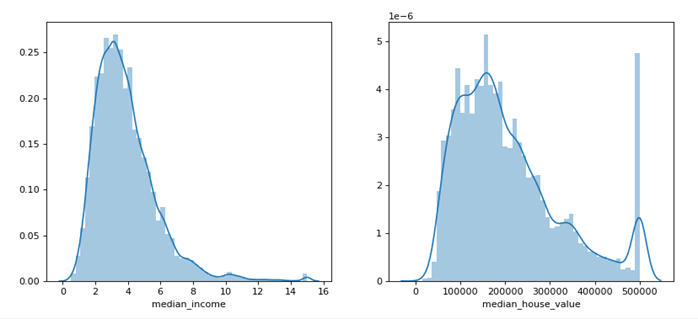5. 俘获异常值
# 定义一个俘获异常值的函数
# 根据正态分布的特性，可以将3*sigma之外的数据视为异常值。
def outlierCapturer(col):
mean, std = data[col].mean(), data[col].std()
lower, upper = mean - 3 * std, mean + 3 * std
return data[col][(data[col]<lower) | (data[col]>upper)]

print(len(outlierCapturer("median_income")))
print(outlierCapturer("median_house_value"))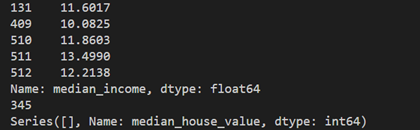可以看到收入中位数的异常值有345条，而房价中位数的分布则没有异常值。这说明该数据集中没有统计学意义上特别便宜和特别贵的房子。

plt.figure(figsize=(12, 5), dpi=80)
#第一行第一列图形
ax1 = plt.subplot(1,2,1)
#第一行第二列图形
ax2 = plt.subplot(1,2,2)

#选择ax1
plt.sca(ax1)
sns.boxplot(data=data["median_income"], color="#2b4750")

#选择ax2
plt.sca(ax2)
sns.boxplot(data=data["median_house_value"], color="#dc2624")
plt.show()
从箱线图中可以看到收入中位数有相当多的数据距离整体分布较远，而房价中位数有少量价格大于50万美元的被归为异常值。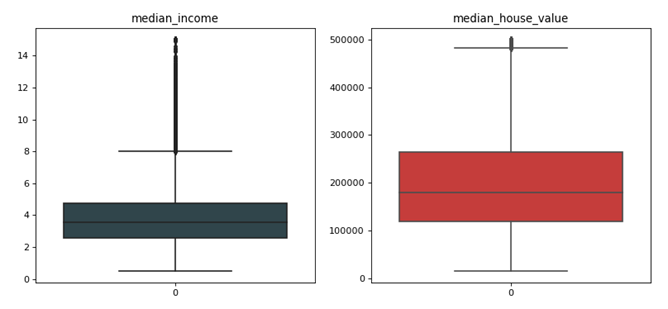6. 对数转换
# 对数转换
fig, ax = plt.subplots(1, 2)
fig.set_size_inches(15,5)
sns.distplot(data["median_income"], ax=ax, color="#2b4750")
sns.distplot(np.log(data["median_income"]), ax=ax, color="#dc2624")
plt.show()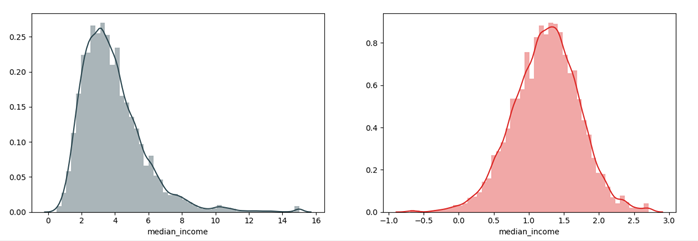可以看到通过对数据取对数，数据的右偏得到了明显的缓解。 7. 寻找重复值 使用DataFrame.duplicated()函数可以查看数据集中的重复值。可以看到该数据集中没有重复的记录。
# 发现重复值
print(data.duplicated().sum())
# 如果有重复值，可以这样查看重复值
# print(data[data.duplicated(keep=False)])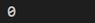8. 绘制seaborn.stripplot图
plt.figure(figsize=(12, 6))
plt.tick_params(labelsize=18)
sns.stripplot(x="ocean_proximity", y="median_house_value", data=data, jitter=True, alpha=0.3)
plt.xticks(fontsize=18)
plt.yticks(fontsize=18)
plt.xlabel('ocean_proximity', {"size":18})
plt.ylabel('median_house_value', {"size":18})
plt.show()
可以看到价格最低的房屋密集分布在内陆，而无论房屋位置在哪里，40万美元以上的房子都属于相对少数。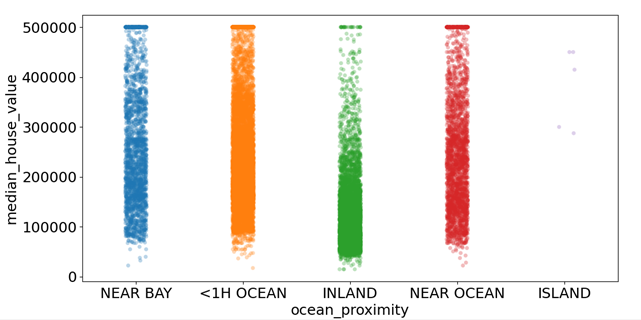9. 房产均价的柱状图
print(data.groupby("ocean_proximity")["median_house_value"].mean())
sns.barplot(x="ocean_proximity", y="median_house_value", data=data)
plt.show()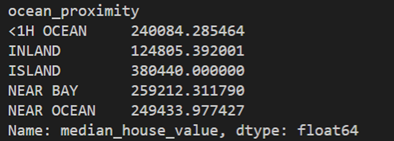根据房产距离海岸的距离将房产分为“岛屿”、“内陆”、“到海岸1小时车程”、“邻近湾区”、“海岸附近”等五类，对着五类求平均值，然后绘制平均值的柱状图。可以看到：岛屿的房产均价最高，房价波动也最大。内陆的房产均价最低，而除了岛屿上的房地产，其他四种的房价波动值都较小。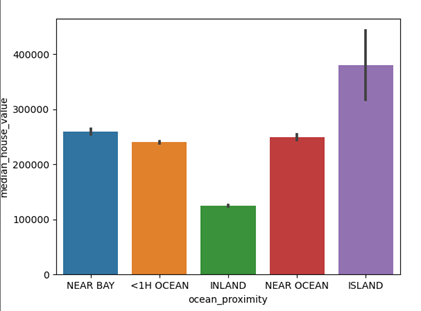10. 绘制经纬度图
fig, ax = plt.subplots(1,2)
plt.figure(figsize=(12, 8), dpi=80)

data.plot(kind="scatter", x="longitude", y="latitude", ax=ax, color="#2b4750")
data.plot(kind='scatter', x='longitude', y='latitude', ax=ax, color="#2b4750", alpha=0.1)
data.plot(kind='scatter', x='longitude', y='latitude',  ax=ax, s=data['population']/100, label='Population', alpha=0.4)
plt.show() 
可以看到中间的图可以清楚地看到房地产分布的高密度地区，这些地区集中了大湾区，洛杉矶和圣地亚哥等经济发达区域。而右边的图在经纬度的基础上叠加了人口。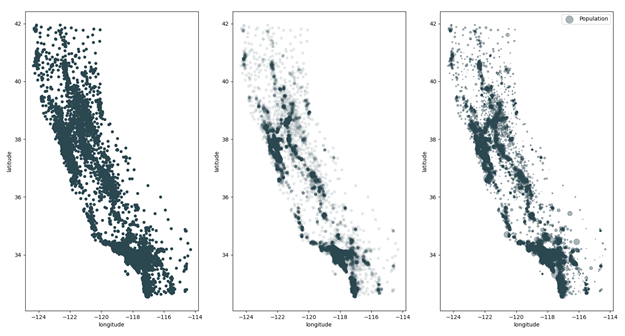11.小提琴图–violinplot 用violinplot可以看到比stripplot更精细的分布。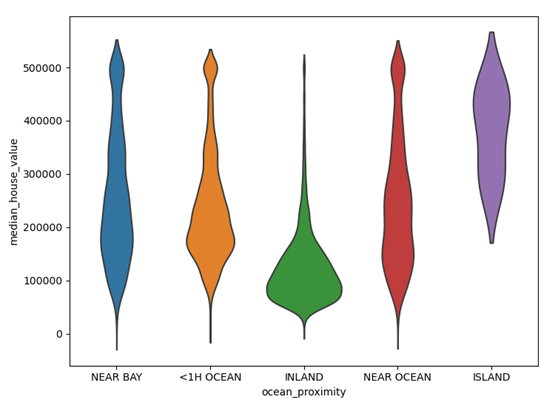12. 查看离岸情况的分布统计 可以看到距离海边小于1小时车程的房产有9136处，而岛屿上的房产只有5处。
print(data["ocean_proximity"].value_counts())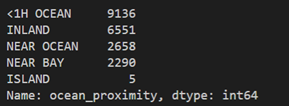13. 使用sklearn的线性回归模型预测房价 我们已经留意到卧室数量属性信息有缺省，因此，我们先用该列的中位数填充这些缺省值。然后用DataFrame.isnull().sum()来统计缺省值的总数。我们用sklearn自带的评分函数lr.score() 对训练结果进行打分。这个函数的返回值中可能得到的最好的分数是1，如果是负值，说明模型变得更加糟糕。当一个模型不论输入何种特征值，其总是输出期望的y的时候，此时返回0。可以看到训练集和测试集测试得分分别为0.636和0.629。
from sklearn.linear_model import LinearRegression
from sklearn.model_selection import train_test_split #划分训练集和测试集

# 用中位数填充total_bedrooms属性的缺省值
data.fillna({"total_bedrooms": data["total_bedrooms"].median()}, inplace=True)
print(data.isnull().sum())

X = data.drop(["ocean_proximity", "median_house_value"], axis=1)
y = data["median_house_value"]
X_train, X_test, y_train, y_test = train_test_split(X, y, test_size=0.3, random_state=42)

lr = LinearRegression()
lr.fit(X_train, y_train)
print(lr.score(X_train, y_train))
print(lr.score(X_test, y_test))

y_hat = lr.predict(X_test)
plt.figure(figsize=(12,4))

plt.plot(y_test.values[-200:], "#2b4750", label="Real Value", marker="o")
plt.plot(y_hat[-200:], "#dc2624", label="Estimated Value", marker="D")

plt.legend(loc="upper_left")
plt.title("Estimated values of a Linear Regression Model", fontsize=20)
plt.show()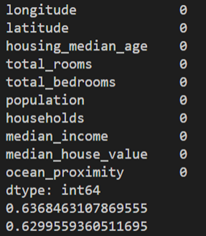然后我们用散点图分别绘制测试集真实值和测试集预测值（代码中的y_hat变量）在数据开头部位，中间部位和尾部200个数据点的可视化情况。可以看到大部分数据吻合的还是较好的，吻合不好的数据主要集中在较高的房价区域。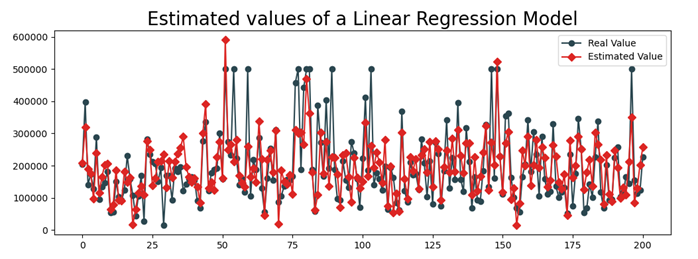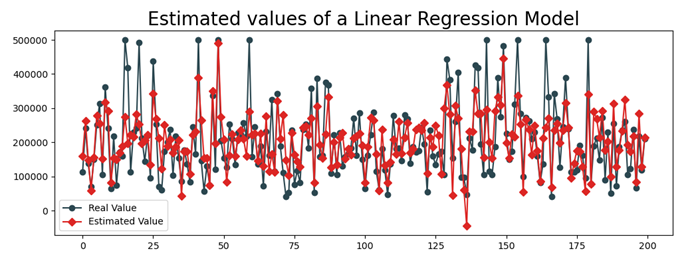展开全文机器学习 python 统计学
• 在市场的经济活动中，经常会遇到某一市场现象的发展和变化取决于几个影响因素的...这时采用一元回归分析预测法进行预测是难以奏效的，需要采用多元回归分析预测法。 　多元回归分析预测法，是指通过对两个或两个以机器学习
• hist = pd.DataFrame(history.history) hist['epoch'] = history.epoch hist.tail()python
• Chilepinos-Wings-线性回归 预测Chiltepinos Wings日销售量的机器学习项目Python
• Python机器学习/数据挖掘项目实战 波士顿房价预测 回归分析python 机器学习 数据挖掘 数据分析 可视化
• 预测区间估计(prediction interval estimate)：利用估计的回归方程，对于自变量 x 的一个给定值 x0 ，求出因变量 y 的一个个别值的估计区间。变量的估计叫预测区间，预测区间反映了单个数值的不确定性； 置信...置信区间
• Python分析51job岗位、多元回归建模预测薪资 ** 项目背景 最近在寻找数据分析师的工作，需要了解数据分析师相关岗位的现状，通过招聘网站信息来了解企业的用人要求是最直接也是最简单的途径，这里以Python岗位为...数据分析 Python
• 逻辑回归是一种广义的线性回归分析模型，通常用于数据挖掘，疾病自动诊断，经济预测等领域。本报告应用python语言中的scikit-learn模块下的逻辑回归模型(LogisticRegression), 对数据集LogiReg_data.csv中记录的100...机器学习 python
• 预测主要是使用回归预测预测结果比较简陋，但是也可以通过这个小项目，来简单地学习一下基于Python的回归预测。 在本文中，我实现了三种回归预测算法： 1. 支持向量回归(SVR) 2. logistic回归 3. 以及使用核...
• 薪水预测：一个使用线性回归和库numpy，pandas，sklearn，matplotlib预测排尿的小型项目Python
• 本资源是基于python实现的Boston Housing 数据集房价预测回归问题，调用了sklearn中5种回归算法对房价进行预测
• 使用随机森林的汽车价格预测：该项目使用随机森林回归器作为监督的机器学习技术来预测汽车价格
• 一个基于python的项目，用于基于RNN和回归预测值 这种方法使用 10 个最相关的股票代码的调整收盘价、道琼斯指数、SnP 500、谷歌趋势、新闻数据等指标和股票市场预测指标，如支撑、阻力、波动率指数和移动平均线，...
• 本资源使用线性回归的手段模拟预测PM2.5，包含了所有的数据以及代码。代码含有详细地注释，欢迎下载学习使用！
• 一、项目背景 北京PM2.5浓度回归分析训练赛 1.数据   数据主要包括2010年1月1日至2014年12月31日间北京pm2.5指数以及相关天气指数数据。   数据分为训练数据和测试数据，分别保存在pm25_train.csv和pm25_test....机器学习
• 还有一种常见的机器学习问题是回归问题，它预测的是连续值而不是离散标签，例如，根据气象数据预测明天气温，或者根据软件说明书预测项目完成所需要的时间。 数据介绍 这里我们介绍一下数据。要预测的是是20...
• 数据集： ...一共取出 240 笔不重复的 test data，请根据 feature 预测这 240 笔的 PM2.5。 数据集下载链接：https://aistudio.baidu.com/aistudio/datasetlist/2 项目代码： from sklearn import linearpython 机器学习 pycharm...